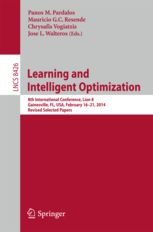• A
• A
• A
• ABC
• ABC
• ABC
• А
• А
• А
• А
• А
Regular version of the site

## The Theory of Set Tolerances

P. 362-377.
Jäger G., Goldengorin B. I., Pardalos P. M.

The theory of single upper and lower tolerances for combinatorial minimization problems has been formalized in 2005 for the three types of cost functions sum, product and maximum, and since then shown to be rather useful in creating heuristics and exact algorithms for the Traveling Salesman Problem and related problems. In this paper for these three types of cost functions we extend this theory from single to set tolerances and the related reverse set tolerances. In particular, we characterize specific values of (reverse) set upper and lower tolerances as positive and infinite, and we present a criterion for the uniqueness of an optimal solution to a combinatorial minimization problem. Furthermore, we present formulas or bounds for computing (reverse) set upper and lower tolerances using the relation to their corresponding single tolerance counterparts. Finally, we give formulas for the minimum and maximum (reverse) set upper and lower tolerances using again their corresponding single tolerance counterparts.

### In bookVol. 8426. Springer, 2014.